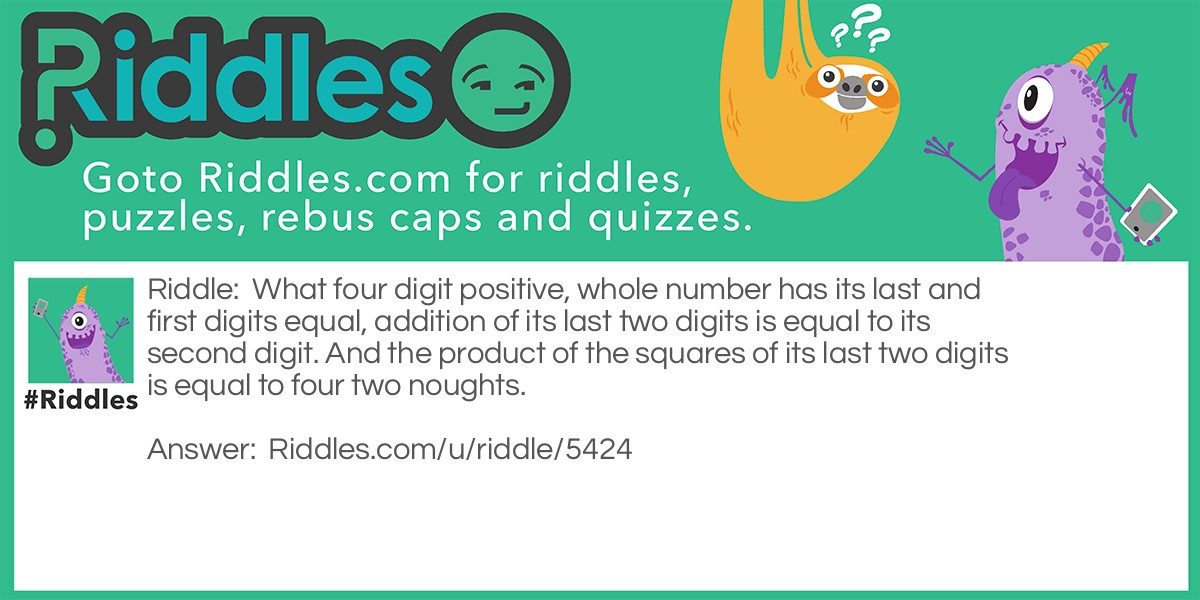# What Four Digit Positive, Whole Number Has Its Last And First Digits Equal...

Author: Moroyei Bayelsa Ebiakpo
6 years ago

Riddle: What four digit positive, whole number has its last and first digits equal, addition of its last two digits is equal to its second digit. And the product of the squares of its last two digits is equal to four two noughts.
Answer: 5945. 5=5. 4+5=9=second digit 4^2 x 5^2 = 400 = four two noughts
VOTE
Source: https://www.riddles.com/5424What four digit positive, whole number has its last and first digits equal... by Moroyei Bayelsa Ebiakpo v1.